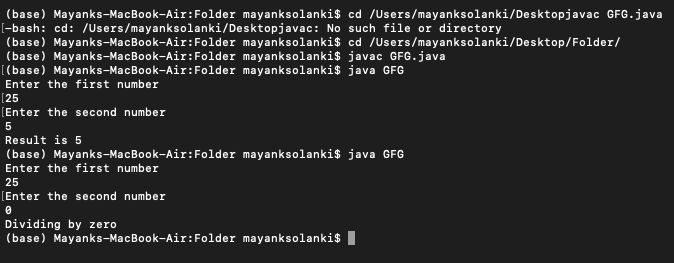Java Program to use Catch to Handle the Exception

• Last Updated : 11 Nov, 2020

The exception is the event occurs when the program is executing. Due to this exception, the normal flow of the program will get disrupts. Whenever an exception occurs in the method, the method creates an object and sends that object to the runtime system. For example, the file needs to be open is not found, class not found exception, Arithmetic Exception, SQL Exception, etc.

To handle these exceptions there are 4 standard techniques:

• Try-catch
• Throw
• Throws
• Using HandlerExceptionResolver interface (Only in Spring-framework)

Catch usage is described here below:

Syntax:

try {

// Put the code in the try block which may occur any
// kind of exception
}
catch (ExceptionName e) {

// handle the exception
}

There are two examples discussed below:

1. Using predefined exception Class
2. Using defining own exception Class

Example 1: Usage of catch with a predefined exception class

This is an example of a predefined exception which is handled by try and catch block.

Java

 // Importing Classes/Filesimport java.io.*;import java.util.*;  // Importing Scanner Class for user inputimport java.util.Scanner;  class GFG {        // Main driver method    public static void main(String[] args)    {        // Taking input from user        Scanner input = new Scanner(System.in);          // Taking input of first number        System.out.println("Enter the first number");        Integer number1 = input.nextInt();          // Taking input of second number        System.out.println("Enter the second number");        Integer number2 = input.nextInt();          // Considering values in order to show execution        // number1 = 30; number2 = 10;          try {              // Dividing both the numbers            int result = number1 / number2;              // If number2 = 0, method will create the object            // of ArithmeticException class and throw it            System.out.println("Result is " + result);            // Printing the result        }          // If there is any  exception in the try block        // then catch will handle the exception        catch (ArithmeticException e) {              // Message printed after catching the exception            System.out.println("Dividing by zero");        }    }}

Output:Example 2: Usage of catch by defining the exception class

In this example, the exception is user-defined in which exception class is written as follows:

Java

 // Java Program to Use catch to handle the exception  // Importing generic Classes/Filesimport java.io.*;import java.util.*;  class GFG {      // Main driver method    public static void main(String[] args)    {          // Taking input from keyboard/user        Scanner input = new Scanner(System.in);          // Enter any name        System.out.println("Enter the name");        String name = input.next();        try {            // Enter age            System.out.println("Enter the age");              // Using nextInt function to read integer            Integer age = input.nextInt();            if (age <= 18) {                MyException me = new MyException();                throw me;                // If age is less then equal to 18 then the                // object of MyException class will be throw                // here            }            else              // If age is greater then 18 i.e no exception is            // there then try block will execute            {                System.out.println("name:" + name);                System.out.println("age:" + age);            }        }        catch (MyException e)        // If the exception will occur then the object that        // throw in the try block will be copied in to this        // object        {            System.out.println(e);        }    }}// User defined MyException classclass MyException extends RuntimeException {    public String toString()    {        // toString method will get Override here        return "Age must be greater then 18";    }}

Input 1:
Enter the name : Geek
Enter the age: 18

Output:
Age must be greater then 18  // Age is equal to 18 so the exception is occured

Input 2:
Enter the name : Geek
Enter the age: 20

Output:
name: Geek
age:20                      // Age is greater then 18 so no exception.

My Personal Notes arrow_drop_up